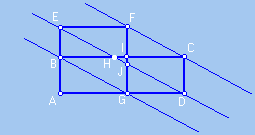### Demonstration that the rectangle ABCD is scissors congruent to the square AEFG.

We overlap the square to the rectangle and trace straight lines FC, ED and BG. Our main objective is to demonstate the congruence of the pairs of triangles GDJ and BHE, and DCH and JFE.
As we are supposing that the base of the rectangle is less than twice the base of the square, straight line ED intercepts the side of the square in the interior of the rectangle.

The triangles which we want to show congruent, are all of the right triangles. Visually we have in the figure diverse parallelograms, which go in guaranteeing congruences to them between legs of the triangles. Thus it is enough to show that  these parallelograms exist.

Of the equality of areas we have AB.AD = AE.AG, and therefore AD/AG=AE/AB.
From this we conclude that straight lines BG and ED are parallel segments, and
therefore BEJG and GBHD are parallelograms ,
using here the characterization of parallelogram in terms of parallel opposite sides.
Also we have that FJDC is parallelogram.In fact: Since BEJG is a parallelogram, we have congruent sides EB and JG, and as EB is congruent to FI it follows, from transitivity, that FI is congruent to  JG. We have then FJ congruent to IG, and consequently, is congruent to CD, which allows one to conclude that FJDC also is parallelogram , using here the characterization of parallelogram in terms of pair of parallel and congruent opposite sides.
Since FJDC is a parallelogram we conclude that straight lines ED and FC are parallel segments, and therefore EFCH also is parallelogram having pairs of parallel opposite sides.

From the existence of these four parallelograms we conclude that the segments are congruent:
* GD and BH, BE and GJ, and therefore  triangles GDJ and BHE
are congruent;
* DC and JF, CH and FE, and therefore  DCH and JFE
are congruent triangles.